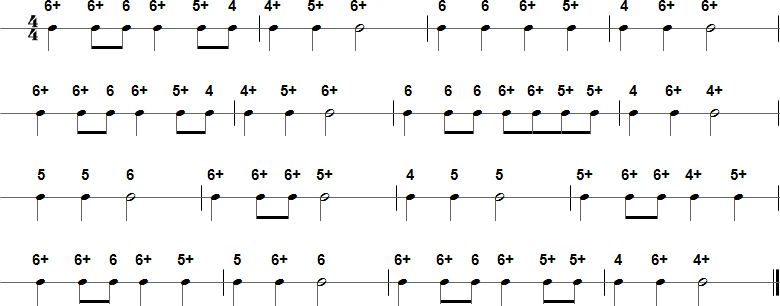Browse by Title:

# Up on the Housetop - Harmonica Tablature and Sheet Music## Details

• Title(s): Up on the Housetop
• Composer: Benjamin Hanby
• Category: Christmas Songs

## Tab in Text Format

6+ 6+ 6 6+ 5+ 4 4+ 5+ 6+ 6 6 6+ 5+ 4 6+ 6+ 6+ 6+ 6 6+ 5+ 4 4+ 5+ 6+ 6 6 6 6+ 6+ 5+ 5+ 4 6+ 4+ 5 5 6 6+ 6+ 6+ 5+ 4 5 5 5+ 6+ 6+ 4+ 5+ 6+ 6+ 6 6+ 5+ 5 6+ 6 6+ 6+ 6 6+ 5+ 5+ 4 6+ 4+